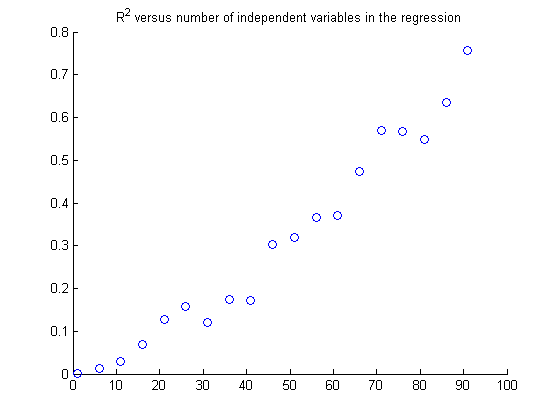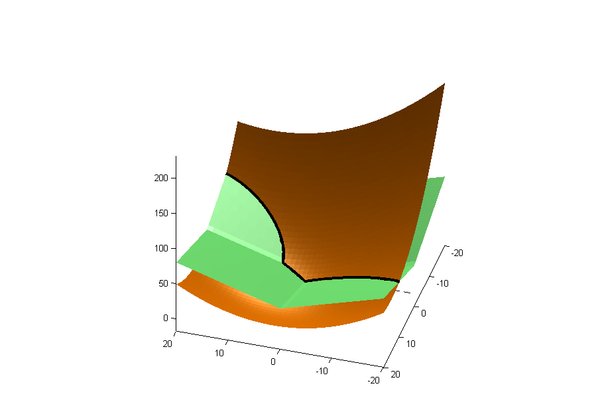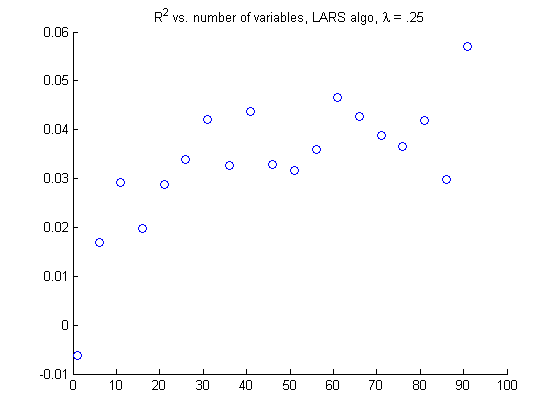# Least Squares Regression with L1 Penalty

Posted by Cameron Davidson-Pilon on

I'd like to discuss and demonstrate a really cool idea in machine learning and statistics. It's a simple idea: adding a constraint to an optimization problem, specifically a constraint on the sum of learned parameters, can have huge impacts on your interpretation, robustness and sanity. I must first introduce the family of functions we will be discussing.

### The L-norm family

The family of L-norm penalty functions, $$L_p:R^d \rightarrow R$$, is defined: $$L_p( x ) = || x ||_p = \left( \sum_{i=1}^d |x_i|^p \right) ^{1/p} \;\: p>0$$ For $$p=2$$, this is the familiar Euclidean distance. The family of functions defined by $$(L_p)^p$$ are often used in machine learning literature. For example, one of my favourite ideas in regression and optimization is using an L-norm function in your optimization problem. For example, in linear regression one might use the following objective function: $$\; \min_w (Y - Xw)^{T}(Y-Xw) + \lambda ||w||_2^2$$ $$= \min_w (Y - Xw)^{T}(Y-Xw) + \lambda w^Tw$$ The above optimization problem is also known as ridge regression in literature. This is a well defined, convex, differentiable optimization problem even after including L2 function. The L2 function acts as a penalizer: $$w^Tw$$ grows large as the weights $$w$$ grow large, thus the minimization program tries to make $$w$$ smaller. Hence why it is called a penalty function. Why is having smaller weights desirable? Smaller weights mean over-fitting is more difficult to achieve.

### Issues with Ridge Regression

One major issue I have with ridge regression, including non-penalized least squares (which is ridge regression when $$\lambda=0$$), is that the solution will give non-zero weights to all variables, even variables known to be completely independent of the response variable! Admittedly the coefficient might be magnificently small, it will never be zero. Example (in Matlab):

>> y = normrnd(5,.1, 1,100);
>> x =  normrnd(5,.1, 1,100);

>> regress( y, [x', ones(100,1)] )
ans
= [-0.0439, 5.2328];


So least-squares has given a non-zero weighting to a completely-independent variable. Of course, one can perform statistical tests to determine whether the regression is significant, or examine the $$R^2$$ to see whether the relationship is linearly-strong. But this is a simple 1-D case. When we are dealing with many variables, the techniques to determine significance are not so simple: one cannot be sure about the relationship between variables, so standard tests are much more complex. Furthermore, we would like a simple solution, that is, a regression result without too many variables. That way it is easier to explain the relevant variables in the model. (Imagine trying to explain to your boss that your analysis using least squares implies that there is a linear relationship between the stock price movements and this computer-generated random variable!) Even more disheartening: we can throw in as many independent variables as we like and still achieve a very high $$R^2$$, see below:

R = []
y =  normrnd(5,.1, 1,100)';
for i=1:5:95
x = normrnd(5,.1, i,100)'; #generate (i,100) random normal variables
[beta, b, r] = regress( y, [x, ones(100,1)] ); #regress on y
R(end+1) = 1 - std(r)/std(y); #calculate R^2 using the residuals, r
end

scatter( 1:5:95, R) #shown below.Wow. I am saddened about this. What if after regression we just removed all variables with a small coefficient? This Cross-Validated answer explains why this is a dangerous idea. What is needed is a new approach.

### Least Squares Regression with L1 Penalty

We make a slight modification to the optimization problem above and big things happen. Instead of using an L2 penalization function, we instead use an L1. $$\; \min_w (Y - Xw)^T(Y-Xw) + \lambda ||w||_1$$ $$= \min_w (Y - Xw)^T(Y-Xw) + \lambda \sum_{i=1}^n | w_i |$$ Immediately we lose the differentiability of the optimization function: the absolute values are non-differentiable. Thus we cannot solve the optimization problem using standard calculus techniques. What do we gain then? One can show that for some large enough $$\lambda$$, the solution is a sparse solution. A sparse solution means a solution for $$w$$ that has many zeros in it, effectively removing the corresponding variable from the system. There are then two immediate consequences:

1. The system will give a coefficient of zero to non-relevant variables, instead of some insanely small coefficient. This means independent or near-independent variables will be discarded instead of included.
2. It gives us the most important variables in the regression model! We can speak confidently that variable $$i$$ has a strong connection with the response variable, $$Y$$ .

We can actually rewrite the above optimization program into a more understandable form. It can be show that the program is equivalent to: $$\min_w (Y - Xw)^T(Y-Xw) \;\; \text{s.t}$$ $$\sum_{i=1}^n | w_i | \le 1/ \lambda$$ So we constrain the sum of the weights. It is interesting to note that as $$\lambda \rightarrow 0$$, we recover the standard Least-Squares solution. Using the figures below, we can try to visualize why the system gives a sparse solution. Consider a regression with two variables, and no linear intercept: $$\min_{w_1, w_2} F(w_1, w_2) + \lambda ||w ||_1$$ where $$||w||_1 = ( |w_1| + |w_2| )$$ and $$F$$ is some quadratic function of $$(w_1, w_2)$$(Recall the term $$(Y-Xw)^{T}(Y-Xw)$$ is quadratic in $$w$$). Visually, the two functions look like:The green surface is $$|w_1| + |w_2|$$ and the gold surface is the quadratic $$F$$. The bold curve represents the curve of intersection. The minimum of the optimization function will lie somewhere on the curve of intersection. If we examine the contour plot of the two functions, including the line of intersection, we see that the minimum does indeed lie on one of the two axes. Also note the sharp breaks in the line of intersection, hence our loss of ability to find the solution using calculus.

### A worked example

#### The dataset and results

Let's compare the coefficients of L1 versus unpenalized linear regression . I used the Death Rate dataset, which uses the following variables to predict regional death rates (emphasis added for effect):

• A1, the average annual precipitation;
• A2, the average January temperature;
• A3, the average July temperature;
• A4, the size of the population older than 65;
• A5, the number of members per household;
• A6, the number of years of schooling for persons over 22;
• A7, the number of households with fully equipped kitchens;
• A8, the population per square mile;
• A9, the size of the nonwhite population;
• A10, the number of office workers;
• A11, the number of families with an income less than \$3000;
• A12, the hydrocarbon pollution index;
• A13, the nitric oxide pollution index;
• A14, the sulfur dioxide pollution index;
• A15, the degree of atmospheric moisture.

After normalizing the data, we perform unpenalized linear regression and L1 linear regression for varying values of $$t = 1/\lambda$$. Below are the coefficients for each regression:

Variable unpenalized LR L1-penalty, $$t=0.2$$ L1-penalty, $$t=1$$ L1-penalty, $$t=1.5$$ L1-penalty, $$t=2$$ L1-penalty, $$t=3$$ L1-penalty, $$t=10$$
A1 0.3326 0 0.1483 0.1977 0.2438 0.3080 0.3326
A2 -0.4192 0 -0.0688 -0.2106 -0.2824 -0.3498 -0.4192
A3 -0.2171 0 0 -0.0230 -0.1214 -0.1787 -0.2171
A4 -0.3306 0 0 0 -0.0233 -0.1770 -0.3306
A5 -0.2510 0 0 0 -0.0507 -0.1614 -0.2510
A6 -0.3295 -0.0161 -0.1731 -0.1721 -0.1821 -0.2361 -0.3295
A7 -0.0947 0 0 -0.0436 -0.0903 -0.1156 -0.0947
A8 0.2347 0 0.0235 0.1388 0.1898 0.2107 0.2347
A9 0.5066 0.1839 0.4030 0.5087 0.5956 0.5588 0.5066
A10 0.0389 0 0 0 -0.0053 -0.0017 0.0389
A11 0.0179 0 0 0 0 0 0.0179
A12 -1.3145 0 0 0 0 -0.2539 -1.3145
A13 1.3909 0 0 0 0 0.2789 1.3909
A14 -0.0351 0 0.1833 0.1965 0.1910 0.1418 -0.0351
A15 0.0468 0 0 0.0088 0.0241 0.0275 0.0468

#### Analysis

Note how as we increase $$t$$, we arrive at the unpenalized least squares solution. This is because we have given so much wiggle room to what the sum of absolute values can be that it is not longer a constraint on the problem. Based on the above results, what can we say about the the coefficients? We can see that there are a handful of variables that are not important in the regression. For example in the $$t=1$$ column, because the respective coefficients are zero we see that the average July temperature, percent of pop. > 65yo, percent of non-white families and relative humidity were chosen not to be included in the regression, as they likely contribute nothing (more accurately: some other variable explains the response variable, or itself, better. For example, variables A6 and A7 are highly correlated, thus why included both in the regression if having either explains most the the pair's contribution to the death rate). It's useful to really scale back $$t$$, which I did by setting $$t = 0.2$$, even if it destroys your predictive power, to see what remains. What does remain should therefore be really important: schooling for persons over 22 and percent of non-white families respectively. Can we try to explain why?

### Back to L1 Penalties

The next question is how does one compute the solution to the above L1 penalized optimization problem? There are three main algorithms that achieve this: Least Angle Regression, LASSO, and stage-wise progression. All have there pros and cons, though for first timers I would suggest LARS (a good MATLAB implementation is available online). Secondly, how does one choose a good $$t$$? Cross validation is a good start. There is no hard set rule, as it is a function of the number of variables in your regression.

We saw previously that by increasing the number of variables in the regression, even if they are independent of the response, we can achieve a high $$R^2$$ with linear regression. What happens if we perform the same test with an L1 penalizer? Well I performed the same test (for science!) and the results are charted below.Fuck yea.

### Conclusion

Often we want our solution to be sparse. Situations may include:

• Suppose you have a basket of financial assets, and you wish to hedge (or replicate) one of them against the others. Normally one might use least-squares to find a really good hedge. But if your basket is large, you will have to trade all of them, even the assets that are highly uncorrelated. This induces more risk and higher transaction costs in practice. Instead, one can use an L1 penalizer with least squares to find the appropriate sub-basket of assets that still gives a really good hedge, and reduces the number of assets to trade.

L1 penalizations are a great way to both better explain your regression results and find important (i.e. abandon non-relevant) features. I have been having a lot of fun playing around with least squares with an L1 penalty. Extensions of this idea to PCA means that we can find a sparse solution to that to, something I am very interested in.

### References

• Tibshirani, Robert. "Regression shrinkage and selection via the lasso." Journal of the Royal Statistical Society. Series B (Methodological) (1996): 267-288.

## Latest Data Science screencasts available

• Hi Cameron, I find this very useful that you have worked out all the details on real data and explain how both the L1 and L2 penalties interact with the squared error term.
However, I don’t understand why “The minimum of the optimization function (quadratic f (w) + L1) will lie somewhere on the curve of intersection.” It seems more like where the gradient of f(w) is the negative of sign(w) defining the quadrant/octant … If this intuition were true in higher dimensions (that the min is when one of the w = 0, say w_3 = 0) then recursively we would conclude that the min is at the global min of minimizing along the individual axes! Plus the intuition seems independent of the strength of t.
Does the following make sense: with an L2 penalty, convergence when it is close to the minimum will be slow (all gradients will be small, by definition of the extremum); whereas with L1 penalty the magnitude of the gradient is constant leading to faster convergence.
Thanks, Ranjeet

Ranjeet Tate on
• Thanks for that!

lucas on
• Hello Cameron, firstly I want to say thank you for writing this post. I found it very useful and well written. I just have a quick question about the dimensions of your inputs in the ridge regression above. You have that y and x are both 1×100 vectors, how is this possible? x is acting as your matrix here right? And then how do you find that w is a 2 dimensional vector?

Thank you so much,

vittorio

vittorio bisin on
• Very nice tutorial dude. Just one point I want to mention is when t=1 feature A9 percent of non-white families has non zero coeff of 0.4, I think it needs to corrected. But thanks cleared so much of mu doubts.

Vinay on